Checkout JEE MAINS 2022 Question Paper Analysis : Checkout JEE MAINS 2022 Question Paper Analysis :

# Conditions of Equilibrium

## What does Static Equilibrium mean?

The word equilibrium implies balance. In particular, static equilibrium suggests that a system is stable and at rest. However, to be completely balanced, the net torque must also be zero.

### Static Equilibrium Example

Consider the Free Body Diagram (FBD) in the given figure.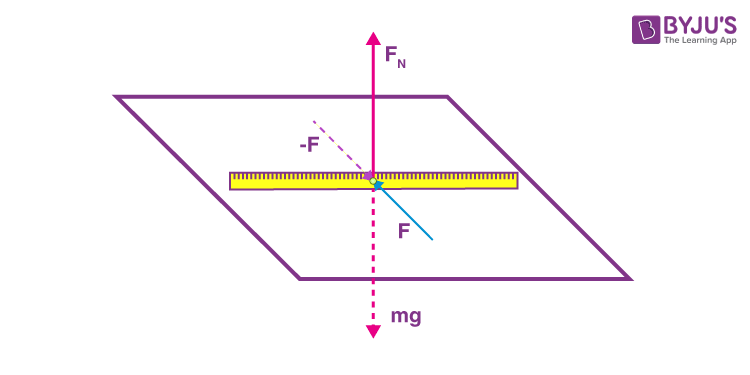We see that a stick is lying on a smooth flat surface with two equal and opposite forces acting upon it. It is evident that the horizontal forces ∑Fx= Fnetx= 0 and the vertical forces ∑Fy = Fnety = 0, and hence, the stick is either at rest or moving with constant velocity.

Now, we apply the forces to the ends of the stick.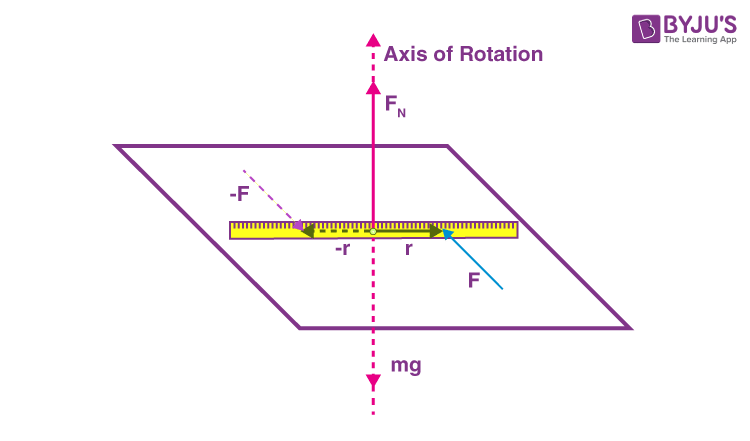It can be seen from the figure that the forces −F and F will make the stick rotate with an angular velocity since both forces act to rotate the meter stick in the same counterclockwise direction. The stick has a net torque working on it.

Just as an object in translational equilibrium can be at rest or moving with constant translational velocity, an object in rotational equilibrium can also rotate with constant angular velocity. This article will focus only on the static equilibrium and the conditions to be satisfied to achieve static equilibrium.

## What are the conditions for a body to be in Static Equilibrium?

Two conditions of equilibrium must be satisfied to ensure that an object remains in static equilibrium. Firstly, the net force acting upon the object must be zero. Secondly, the net torque acting upon the object must also be zero. In other words, both static translational and static rotational equilibrium conditions must be satisfied.

### First Condition of Equilibrium

The first condition of equilibrium states that for an object to remain in equilibrium, the net force acting upon it in all directions must be zero.

The above statement simply means that the body must not be experiencing acceleration. In the form of an equation, the first condition of equilibrium is denoted as follows:

Fnet = 0

The above condition is true for both static equilibrium, where the object’s velocity is zero, and dynamic equilibrium, where the object moves at a constant velocity.

In the picture below, we see a motionless person in static equilibrium. As you can see from the image, the forces acting on him add up to zero.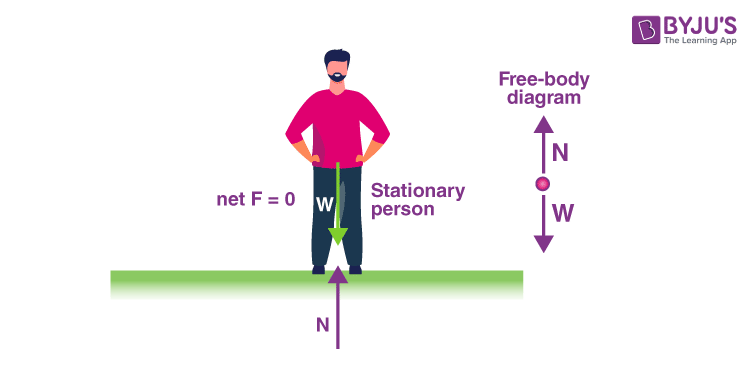In the image below, we see that the car is in dynamic equilibrium as it moves at a constant velocity. There are horizontal and vertical forces, but the net external force in any direction is zero.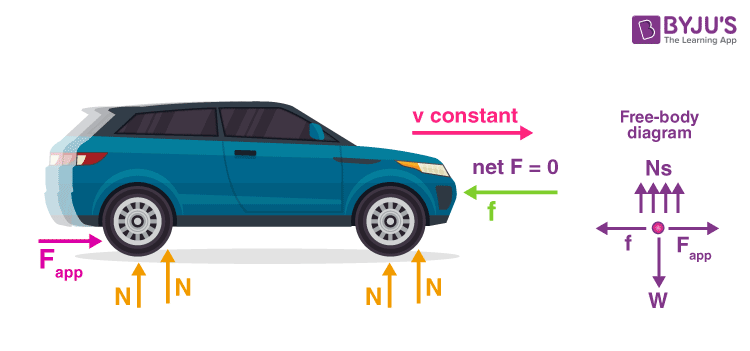## Second Condition of Equilibrium

The second condition of equilibrium states that the net torque acting on the object must be zero.

If the body does not achieve equilibrium even though the first condition for equilibrium is satisfied, it is because it tends to rotate. This situation demands another condition in addition to the first condition for equilibrium. A body satisfies the second condition for equilibrium when the resultant torque acting on it is zero. Mathematically it is represented as:

∑ τ = 0

Torque is the rotational equivalent of a force. The magnitude of torque is given by the equation:

τ = rFsinθ

Where τ is the symbol for torque, r is the distance from the pivot point to the point where the force is applied, F is the magnitude of the force, and θ is the angle between the force and the vector directed from the point of application to the pivot point.

Two kids balancing a seesaw satisfy both conditions for equilibrium. In the image, we see the lighter child sitting farther away from the pivot to create a torque equal in magnitude to that of the heavier child.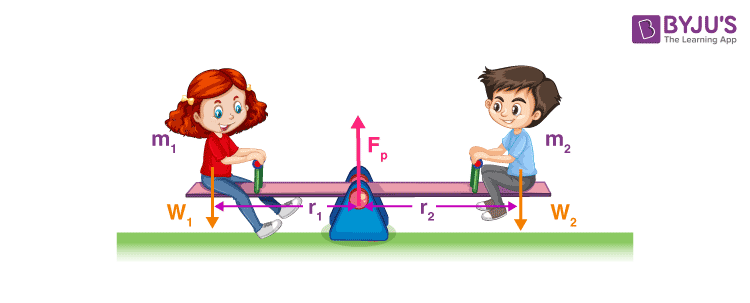## Frequently Asked Questions – FAQs

### What conditions are necessary for static equilibrium?

To achieve complete static equilibrium, a system must possess both rotational equilibrium and translational equilibrium.

### What does Static Equilibrium mean?

Static equilibrium is a term used to suggest that a system is stable and at rest.

### What is a Torque?

Torque is the rotational equivalent of a force. The magnitude of torque is given by the equation:
τ = rFsinθ

### What is the first condition of equilibrium?

The first condition of equilibrium states that for an object to remain in equilibrium, the net force acting upon it in all directions must be zero.

### What is the second condition of equilibrium?

The second condition of equilibrium states that the net torque acting on the object must be zero.

Stay tuned to BYJU’S and Fall in Love with Learning!